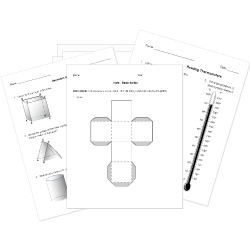Want to see correct answers?Looking for Geometry worksheets?
Check out our pre-made Geometry worksheets!
 Tweet##### Filter By Grade

You are browsing Grade 12 questions. View questions in All Grades.

# Twelfth Grade (Grade 12) Two Dimensional Shapes Questions

You can create printable tests and worksheets from these Grade 12 Two Dimensional Shapes questions! Select one or more questions using the checkboxes above each question. Then click the add selected questions to a test button before moving to another page.

Grade 12 Circles CCSS: HSG-GPE.A.1
What is the equation for a circle with center $(2,5)$ and radius of 5?
1. $(x+2)^2+(y+5)^2=25$
2. $(x-2)^2+(y-5)^2=5$
3. $(x+2)^2+(y+5)^2=5$
4. $(x-2)^2+(y-5)^2=25$
What is the equation for a circle with center $(-4,6)$ and radius of 6?
1. $(x+4)^2+(y-6)^2=12$
2. $(x-4)^2+(y+6)^2=36$
3. $(x+4)^2+(y+6)^2=12$
4. $(x+4)^2+(y-6)^2=36$
Grade 12 Circles CCSS: HSG-GPE.A.1
What is the equation for a circle with center $(2,-2)$ and radius of 2?
1. $(x-2)^2+(y+2)^2=4$
2. $(x-2)^2+(y+2)^2=2$
3. $(x+2)^2+(y-2)^2=2$
4. $(x+2)^2+(y-2)^2=4$
Grade 12 Circles CCSS: HSG-GPE.A.1
What is the equation of a circle with a center of (0, 0) and a radius of 5?
1. $x^2-y^2=25$
2. $x^2+y^2=25$
3. $x^2+y^2=5$
4. $x^2-y^2=5$
Grade 12 Circles CCSS: HSG-GPE.A.1
What is the equation for a circle with center $(-2,-2)$ and the outer point of the radius at $(7,10)$?
1. $(x-2)^2+(y-2)^2=100$
2. $(x+2)^2+(y+2)^2=225$
3. $(x-2)^2+(y-2)^2=289$
4. $(x+2)^2+(y+2)^2=149$
Grade 12 Circles CCSS: HSG-GPE.A.1
What is the equation for a circle with center $(1,-4)$ and the outer point of the radius at $(-3,-5)$?
1. $(x-1)^2+(y+4)^2=15$
2. $(x+1)^2+(y-4)^2=15$
3. $(x-1)^2+(y+4)^2=17$
4. $(x-1)^2+(y+4)^2=289$
Find the center and radius of: $(x-2/3)^2+(y+1/2)^2=9/4.$
1. Center $(-2/3, -1/2), \$ Radius of $3/2$
2. Center $(2/3, -1/2), \$ Radius of $3/2$
3. Center $(2/3, -1/2), \$ Radius of $9/4$
4. Center $(-2/3, 1/2), \$ Radius of $9/4$
$(x-1)^2+(y+2)^2=12$
1. Center (-1,2); Radius of $2sqrt3$
2. Center (1,-2); Radius of $2sqrt3$